### Moment Generalized Maximum Entropy

The default estimation technique is moment generalized maximum entropy (GME-M). This is simply GME with the data constraints modified by multiplying both sides by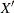. GME-M then becomes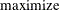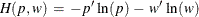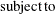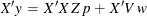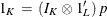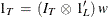There is also the cross entropy version of GME-M, which has the same form as GCE but with the moment constraints.

#### GME versus GME-M

GME-M is more computationally attractive than GME for large data sets because the computational complexity of the estimation problem depends primarily on the number of parameters and not on the number of observations. GME-M is based on the first moment of the data, whereas GME is based on the data itself. If the distribution of the residuals is well defined by its first moment, then GME-M is a good choice. So if the residuals are normally distributed or exponentially distributed, then GME-M should be used. On the other hand if the distribution is Cauchy, lognormal, or some other distribution where the first moment does not describe the distribution, then use GME. See Example 13.1 for an illustration of this point.